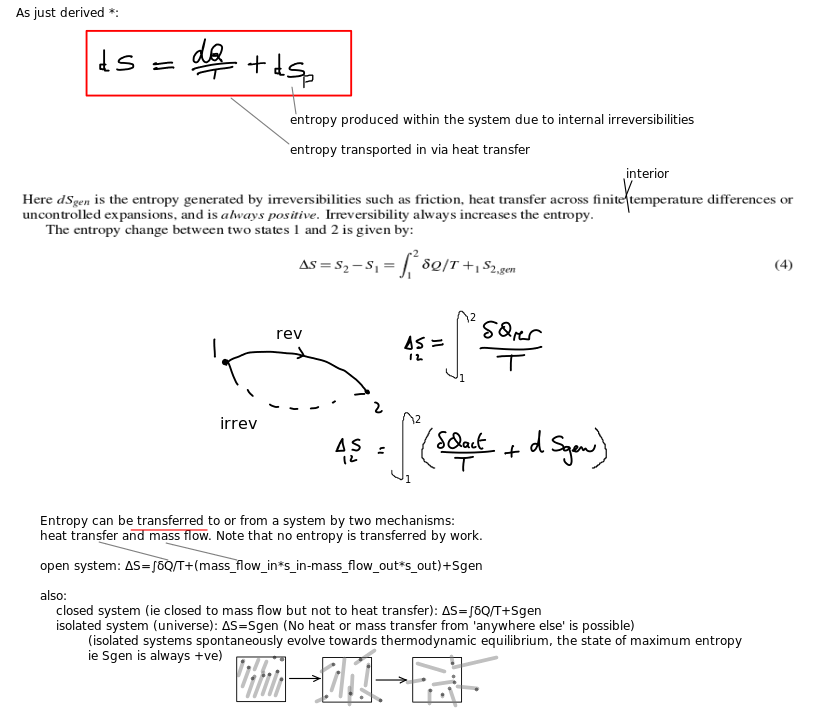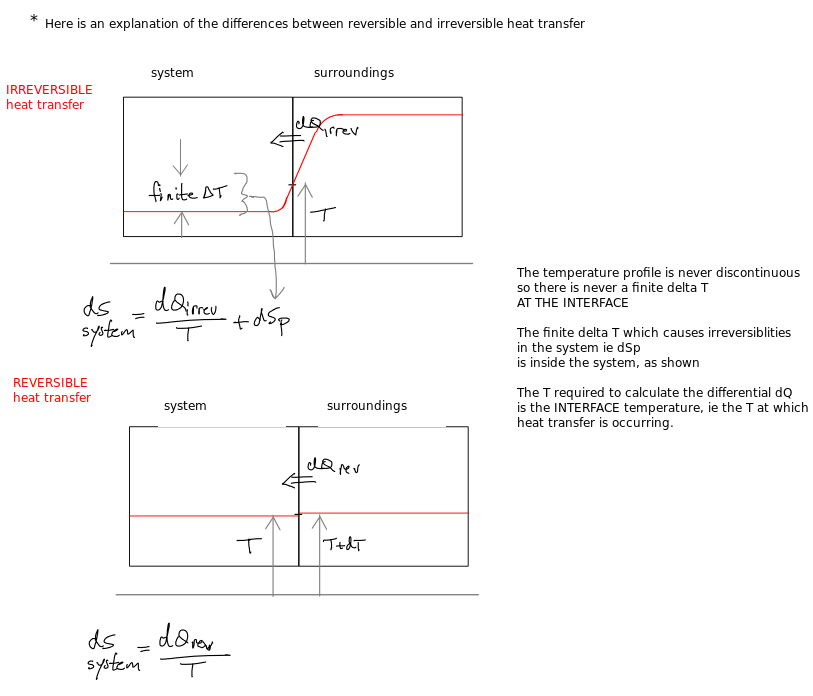## The TdS property relation is always true

Although derived using the idea of a reversible process, this useful relation is applicable to all processes.

## Definition of a reversible processNote here the reference to ‘one-way work transfer’. Work is not a process in itself, but an exchange of energy (which may form part in a process). It therefore makes little sense to talk about reversible or irreversible work. Nonetheless there are recognisable differences between, say, the work that is done by a stirrer and the work that is done by a piston in quasi-equilibrium.

Stirrer work is a transfer of energy which results in dissipation (change of energy from mechanical, bulk or coordinated motion to the thermal (pseudorandom) motion of particles).

Allowing the stirrer freedom to rotate in the opposite direction does not cause energy extraction from the stirred system, however. The stirrer shaft does not spontaneously rotate in the opposite direction and unstir the system contents (hence the term one-way).

Quasi-equilibrium piston work causes zero dissipation. Allowing the piston motion to reverse direction (by reducing the force on the piston to a level infinitesimally less than the force due to internal pressure) does cause the extraction of energy from the compressed system, hence the term two-way work.

I have already talked about the heat transfer aspect of reversibility. The diagram above is correct but does not explain the situation clearly enough.

## How are these 2nd Law equations related to each other?

Sometimes it can be useful to be reminded about the ways in which the various 2nd law equations are interrelated. Here is an outline of my picture of the situation. Other versions of this are of course possible. I just wanted to get my own grip on the logic which links them. It’s worth remembering that the 2nd law is an observation (ie it’s not proved using axioms, despite the earnest hopes of certain physicists).

## What the entropy change equation meansThis is one of those parts of engineering thermodynamics courses which is never made clear enough. The first confusion I suffered from was the idea that entropy increase was always a sign of irreversibility. This is clearly not true, since we can (in theory) increase the entropy of a system just by transferring some heat to it reversibly. Perhaps the single most important thing to remember, however, is that, in the equation for dS, when you transfer heat (dQ) into a system, the local system temperature at the interface is what is meant by T.Now it may be that the rest of the system is also at T, in which case there are no thermal gradients and no additional changes in system entropy. That would be the ideal case of reversible heat transfer (dS=dQ/T). In reality, once the dQ has entered the system, it is faced by some kind of temperature profile (ie the internal T distribution in the system is non-uniform). Any such non uniformities result in non equilibrium conditions. The subsequent passage of heat into the system is therefore irreversible (it’s very hard to keep track of which atoms hit which others and thus how the dissipation process plays out). The extra increase in system entropy which is created, after the effect of transfer of heat in (dQ/T), is summed up as dSgen (aka dSp). This is a term the detailed behaviour of which is hard to account for. It might include the effects of internal friction, pressure gradients etc…anything which represents a deviation from quasi-equilibrium in the system.

It’s quite important to keep a close eye on the language used. I found it easy to get terms like ‘entropy production’ (dSp = dSgen) and ‘entropy increase’ (dS) mixed up.

For a long time, I was also confused by textbooks which said things like “dSgen includes the effects of finite T differences.” So, when you put an object at say 2T in contact with a system at T, did that mean that, in dS=dQ/T +dSgen, the term dQ/T=0 (because the ht was finite-difference and thus irreversible and dSgen included all effects of irreversibility)? Also, what T value was I supposed to use?

I hope it’s clear now that dS=dQ/(interface contact temp) +dSp.